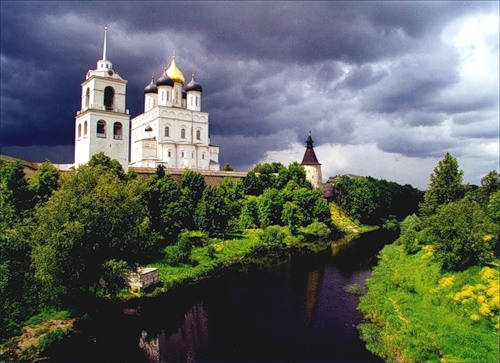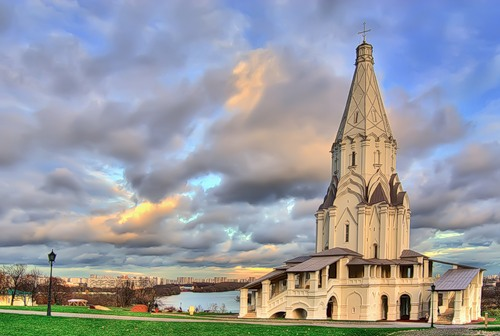Коломенское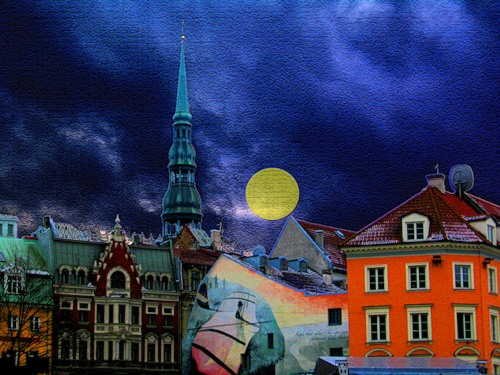Рига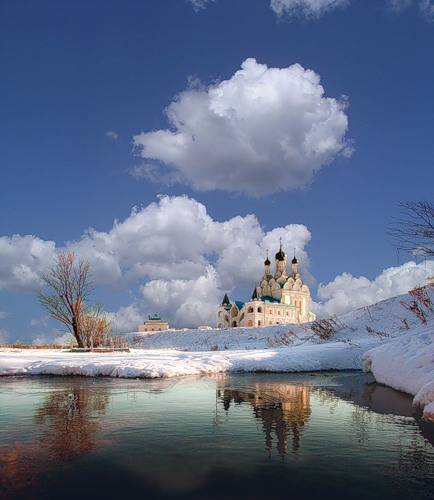храм Благовещения Пресвятой Богородицы, что в селе Тайнинском, около Медведково (Москва).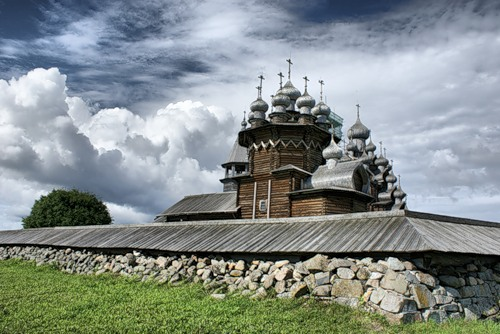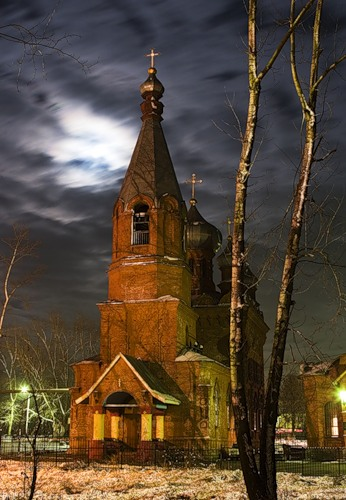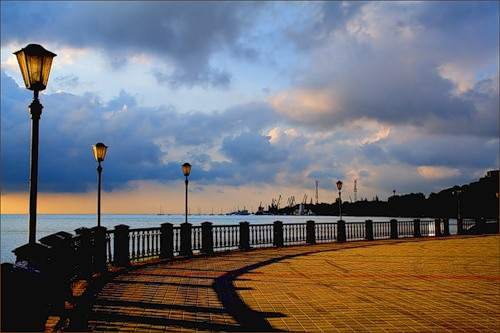Taganrog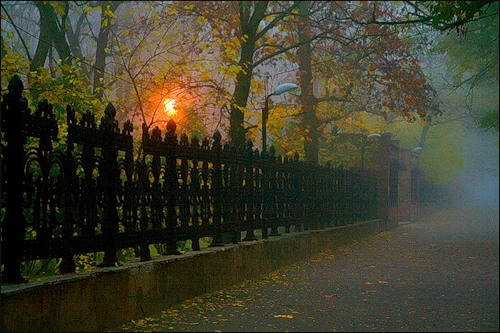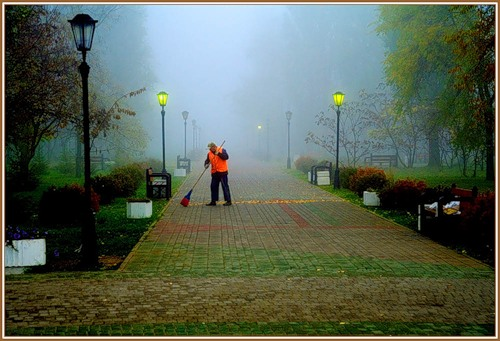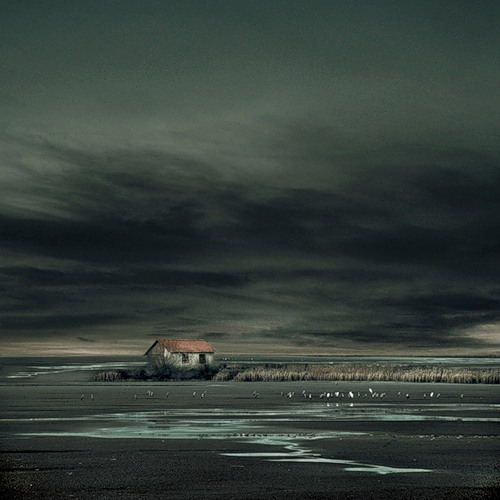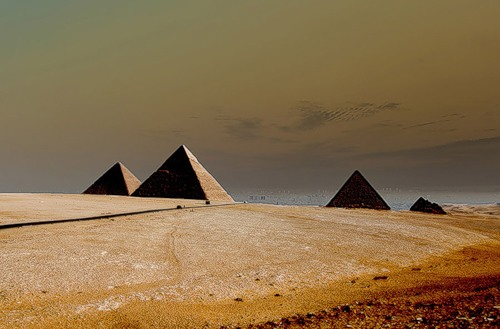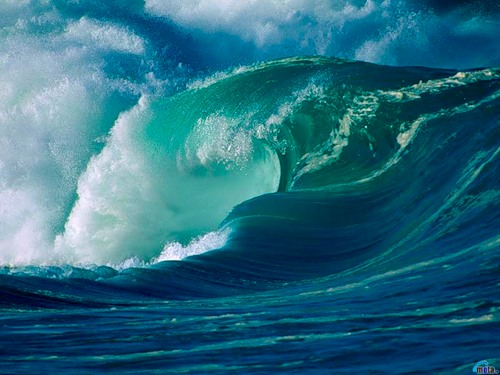Gamma correction, gamma nonlinearity, gamma encoding, or often simply gamma, is the name of a nonlinear operation used to code and decode luminance or tristimulus values in video or still image systems. Gamma correction is, in the simplest cases, defined by the power-law expression
where the input and output values are non-negative real values, typically in a predetermined range such as 0 to 1. A gamma value is sometimes called an encoding gamma, and the process of encoding with this compressive power-law nonlinearity is called gamma compression; conversely a gamma value is called a decoding gamma and the application of the expansive power-law nonlinearity is called gamma expansion

Gamma correction